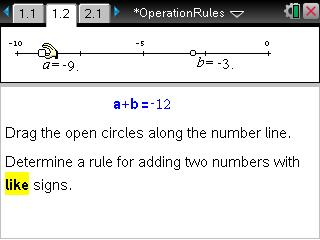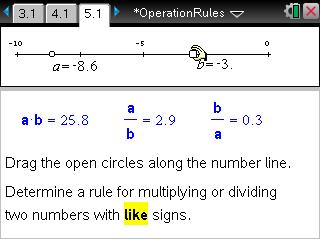# Activities

••• ##### Subject Area

• Math: Algebra I: Equations and Inequalities
• Math: Algebra I: Expressions

• ##### Author9-12

15 Minutes

• ##### Device
•TI-Nspire™ CX/CX II
•TI-Nspire™ CX CAS/CX II CAS
• TI-Nspire™
• TI-Nspire™ CAS
• ##### Software

TI-Nspire™
TI-Nspire™ CAS

3.0

## Operation Rules

#### Activity Overview

In this short activity, students use interactive pages with sliders to determine rules for different combinations of adding and subtracting positive and negative numbers such as adding two negative numbers.

#### Key Steps

•In this short activity, students use interactive pages with sliders to determine rules for different combinations of adding and subtracting positive and negative numbers such as adding two negative numbers.

•In the second problem, students will use the same type of interactive pages to determine rules for different combination of multiplying and dividing positive and negative numbers.